Checkout NEET 2022 Question Paper Analysis : Checkout NEET 2022 Question Paper Analysis :

# Alternating Current formulae for NEET

Alternating current is a current that changes its magnitude and polarity at regular interval of time. If the current maintains its direction constant it is called direct current.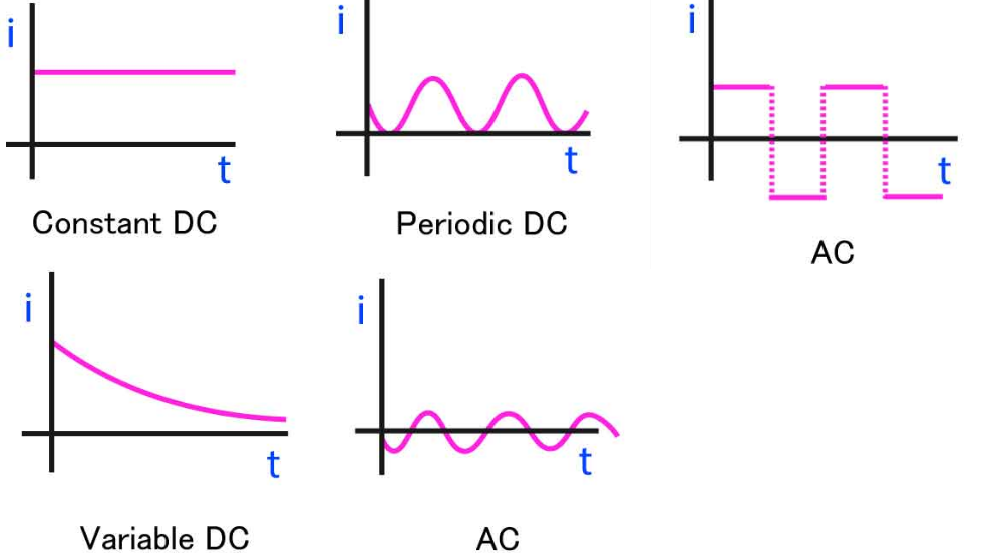AC voltage, v = V0sinωt

AC current, i = I0sinωt

Capacitive Reactance V0/I0 = 1/ωC = Xc

RMS voltage, Vrms = V0/√2

RMS current, Irms = I0/√2

Inductive Reactance  V0/I0 = ωL = XL

Phase angle of an RLC series circuit Φ = tan-1 (XL – XC)/R

AC version of Ohm’s law, I0 = V0/Z

RLC series circuit Impedance ,

$$\begin{array}{l}Z= \sqrt{R^{2}+(X_{L}-X_{C})^{2}}\end{array}$$

Average power associated with circuit element, Pavc = (1/2)I0V0cos Φ

Resonant angular frequency of the circuit, ω0 = √1/LC

Root Mean Square: The Root mean square of a function from T1 to T2 is given by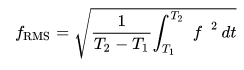Power Consumed or Supplied in AC circuit

The average power consumed in a cycle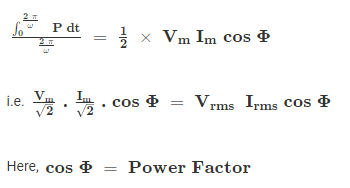Purely Resistive Circuit: In a purely resistive circuit, the power is dissipated by the resistance and phase of both voltage and current remains the same.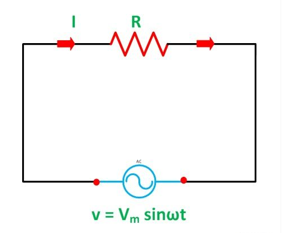P = VrmsIrms cosΦ = Vrms2/R

Pure Inductive Circuit: In a purely inductive circuit current lags the voltage by 900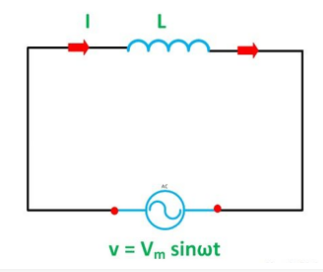i = isin(ωt- π/2)

Purely Conductive Circuit: In a purely inductive circuit, the current flowing through the capacitor leads the voltage by 900.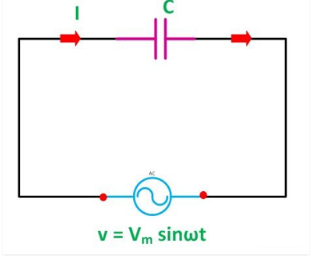I = {Vm/(1/ωC)}cosωt

I = (Vm/Xc)cosωt

I = Imcosωt

I = Imsin(ωt + π/2)

### Recommended Video:

Electric Current and Electrical Networks | Ohm’s Law in Scalar form | NEET 2021/2022/2023 | Physics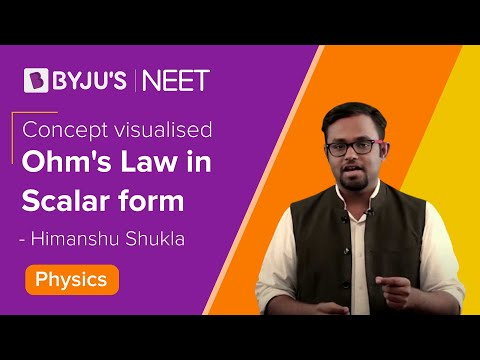You might like: